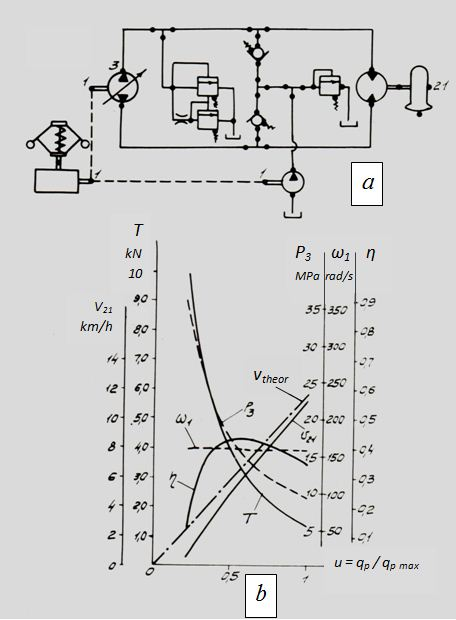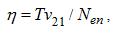Contents >> Analysis and Design >> Systems of Hydraulic Drives >> Hydraulic Drives of Loaders >> Traction analysis of hydraulic transmission of the small-sized loader ТО-31

 Systems of Hydraulic Drives - Traction analysis of the small-sized loader ТО-31 Traction analysis of the small-sized loader ТО-31 Let's consider a problem of traction (static) analysis of volumetric hydraulic transmission of the small-sized loader ТО-31 by means of the program HYSTR .Fig. 1. The transmission fulfilled according to the circuit diagram with closed circulation of a stream (Fig. 1, a ) consists of the diesel engine, the variable displacement reversible pump, the pump of additional charging, the hydraulic motor-wheel, a valve equipment, tees and pipelines. As the volumetric hydraulic transmission is fulfilled according to the onboard diagram and in it except for the diesel engine and systems of additional charging there are two identical closed contours then at condition of rectilinear movement of the machine one onboard hydraulic contour is considered only, and instead of two hydraulic motor-wheels in the rated diagram one is taken with doubling appropriating parameters. For reception of correct results appropriating parameters of the diesel engine and the pump of additional charging are reduced twice (from a condition of half of selected power). Thus «a hook drawn» is equal to traction effort of one board. At transition to the actual diagram the double value of the traction effort takes into account. The rated diagram of volumetric hydraulic transmission (Fig. 1, a ) consists of 27 elements connected in 40 nodes. The order of the solved system of equations of mathematical model is equal 64. Dependence of maximal traction effort Т on parameter of regulation of the basic pump u = q p / q p max , where q p , q p max – current and maximal geometric volume of the basic pump, is as a result received, at a nominal moment on the diesel engine shaft (Fig. 1, b ). As seen from figure, at reduction of regulation parameter u pressure р 3 on the pump output that conforms to growth of «hook» traction effort Т grows. Thus at u = 0.2 speed v 21 of the machine falls practically up to zero; at u = 1 speed v 21 = 12 km/h. The difference between theoretical speed of the machine v theor and actual speed v 21 speaks as follows. At u ≈ 0.2 owing to a high pressure on the basic pump output the volumetric losses depending on pressure, are rather great, therefore a working liquid flow in an input of the hydraulic motor less theoretical, accordingly it is less and speed of the machine. Besides when the parameter u is close to 0.2, value of traction effort comes close to coupling weight and a slipping of wheels occurs. As efficiency of the machinewhere N en – the engine power, at u ≈ 0.2 speed of the machine v 21 is small and η ≈ 0.1 in spite of the fact that Т = 10 kN; at u close to 1, despite of the maximal value of speed ( v 21 ≈ 12 km/h) η ≈ 0.34, as traction effort Т thus falls up to 1.4 kN. The maximal value of efficiency equal η ≈ 0.43, is observed at u = 0.56, v 21 = 6 km/h, Т = 3.4 kN. Carrying out of such calculations on conceptual phase of volumetric hydraulic transmission allows providing on the basis of the multiple analyses of traction characteristics the best schematic design of a drive.

 < Previous Contents Next >